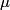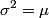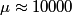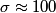## Solution to 1986 Problem 40

 The counts are distributed according to the Poisson distribution. The mean$\mu$ and standard deviation$\sigma$ of a Poisson distribution are related by\begin{align*}\sigma^2 = \mu\end{align*}We take$\mu \approx 10000$ counts/hour which gives$\sigma \approx 100$ counts/hour. Hence, answer (A) is correct.